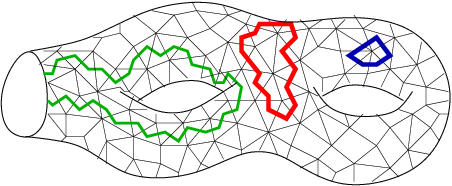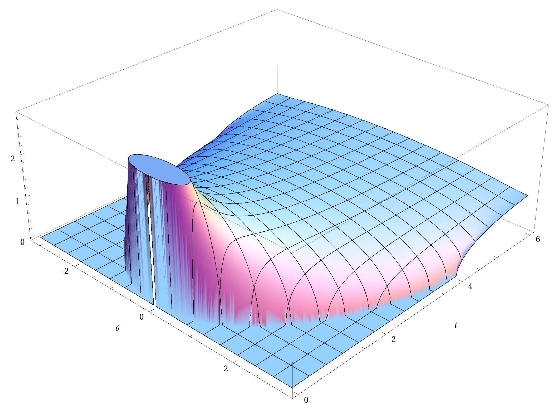# GranMa 2011

## Institut Henri Poincaré, Paris

General

Announcement

Participants

Practical details

Registration

Schedule

Teodor Banica, U Cergy:

## Block-modified Wishart matrices and free Poisson laws

We study the random matrices of type $\tilde{W}=(id\otimes\varphi)W$, where $W$ is a complex Wishart matrix of parameters $(dn,dm)$, and $\varphi:M_n(\mathbb C) \to M_n(\mathbb C)$ is a self-adjoint linear map. We prove that, under suitable assumptions on $\varphi$, we have the limiting $d\to\infty$ eigenvalue distribution formula $\delta m\tilde{W}\sim\pi_{mn\rho}\boxtimes\nu$, where $\rho$ is the law of $\varphi$, viewed as a square matrix, $\pi$ is the free Poisson law, $\nu$ is the law of $D=\varphi(1)$, and $\delta=tr(D)$. This result generalizes all previously known computations. Our assumptions on $\varphi$ are of planar'' type, and we make a number of algebraic speculations in this direction. This is joint work with Ion Nechita.